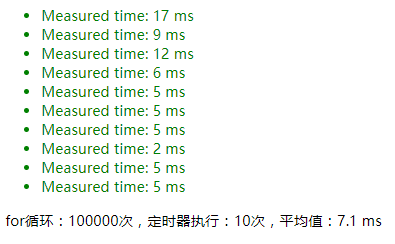# 详解关于JSON.parse()和JSON.stringify()的性能小测试

更新时间：2019年03月14日 09:18:51   作者：ican我要评论

JSON.parse(JSON.stringify(obj))我们一般用来深拷贝，其过程说白了，就是利用 JSON.stringify 将js对象序列化（JSON字符串），再使用JSON.parse来反序列化（还原）js对象。至于这行代码为什么能实现深拷贝，以及它有什么局限性等等，不是本文要介绍的。本文要探究的是，这行代码的执行效率如何？如果随意使用会不会造成一些问题？

```/**
* 一个简单的断言函数
* @param value {Boolean} 断言条件
* @param desc {String} 一个消息
*/
function assert(value, desc) {
let li = document.createElement('li');
li.className = value ? 'pass' : 'fail';
li.appendChild(document.createTextNode(desc));
document.getElementById('results').appendChild(li);
}```
```/**
* 一个测试套件，定时器是为了多次执行减少误差
* @param fn {Function} 需要多次执行的代码块（需要测试、比对性能的代码块）
* @param config {Object} 配置项，maxCount: 执行代码块的for循环次数，times: 定时器执行次数
*/
function intervalTest(fn, config = {}) {
let maxCount = config.maxCount || 1000;
let times = config.times || 10;
let timeArr = [];
let timer = setInterval(function () {
let start = new Date().getTime();
for (let i = 0; i < maxCount; i++) {
fn.call(this);
}
let elapsed = new Date().getTime() - start;
assert(true, 'Measured time: ' + elapsed + ' ms');
timeArr.push(elapsed);
if (timeArr.length === times) {
clearInterval(timer);
let average = timeArr.reduce((p, c) => p + c) / times;
let p = document.createElement('p');
p.innerHTML = `for循环：\${maxCount}次，定时器执行：\${times}次，平均值：\${average} ms`;
document.body.appendChild(p);
}
}, 1000);
}```

```let jsonData = {
title: 'hhhhh',
dateArr: [],
series: [
{
name: 'line1',
data: []
},
{
name: 'line2',
data: []
},
{
name: 'line3',
data: []
},
]
};

let res = [
{
name: 'line1',
value: 1
},
{
name: 'line2',
value: 2
},
{
name: 'line3',
value: 3
},
];```

```/**
* 处理json数据的函数。模拟真实环境中图表数据实时更新
* @param lastData {Object} 上一次的数据
* @param res {Array} 当前数据
* @returns data 处理完成后的结果集
*/
function handleJsonData(lastData, res) {
// 1. 使用 JSON.parse(JSON.stringify()) 深拷贝
let data = JSON.parse(JSON.stringify(lastData));

// 2. 不使用JSON序列化，直接修改参数
// let data = lastData;

if (data.dateArr.length > 60) {
data.dateArr.shift();
for (let i = 0; i < data.series.length; i++) {
data.series[i].data.shift();
}
}
data.dateArr.push(new Date().toTimeString().substr(0, 8));
for (let i = 0; i < data.series.length; i++) {
data.series[i].data.push(res[i].value);
}
return data;
}```

maxCount=100

```let jsonTest = function () {
jsonData = handleJsonData(jsonData, res);
};

intervalTest(jsonTest, {maxCount: 100});```

1.使用 JSON.parse(JSON.stringify()) 深拷贝 的结果：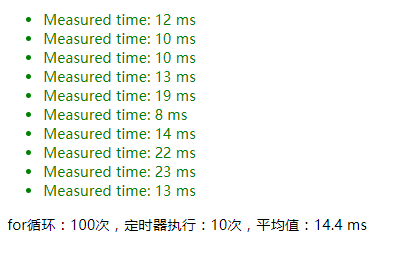2.不使用JSON序列化，直接修改参数 的结果:

```function handleJsonData(lastData, res) {
// 1. 使用 JSON.parse(JSON.stringify()) 深拷贝
// let data = JSON.parse(JSON.stringify(lastData));

// 2. 不使用JSON序列化，直接修改参数
let data = lastData;

// ...
}```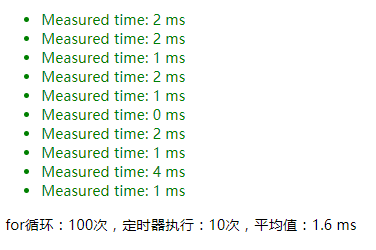maxCount=1000

`intervalTest(jsonTest, {maxCount: 1000});`

1.使用 JSON.parse(JSON.stringify()) 深拷贝 的结果：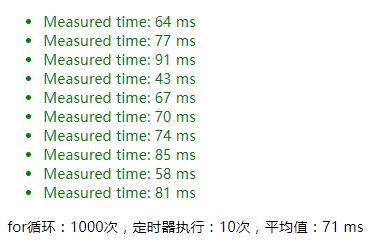2.不使用JSON序列化，直接修改参数 的结果:maxCount=10000

`intervalTest(jsonTest, {maxCount: 10000});`

1.使用 JSON.parse(JSON.stringify()) 深拷贝 的结果：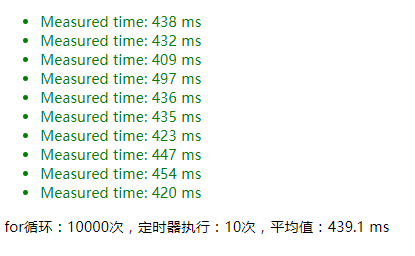2.不使用JSON序列化，直接修改参数 的结果: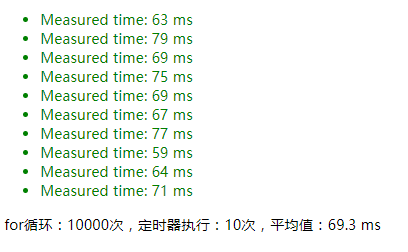```// 1. 使用 JSON.stringify() 判断一个对象是否为空对象
let isEmptyObject1 = function () {
if (JSON.stringify(jsonData) === '{}') {
// do something
}
};

// 2. 使用 Object.keys().length 判断一个对象是否为空对象
let isEmptyObject2 = function () {
if (Object.keys(jsonData).length === 0) {
// do something
}
};```

maxCount=1000

1.使用 JSON.stringify() 判断一个对象是否为空对象 的结果：

`intervalTest(isEmptyObject1, {maxCount: 1000});`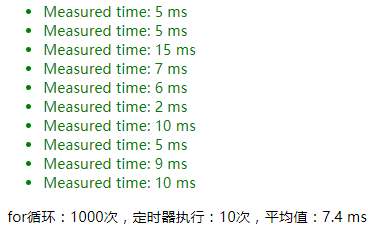2.使用 Object.keys().length 判断一个对象是否为空对象 的结果：

`intervalTest(isEmptyObject2, {maxCount: 1000});`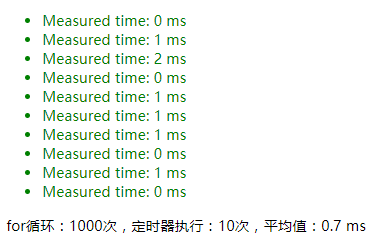maxCount=10000

1.使用 JSON.stringify() 判断一个对象是否为空对象 的结果：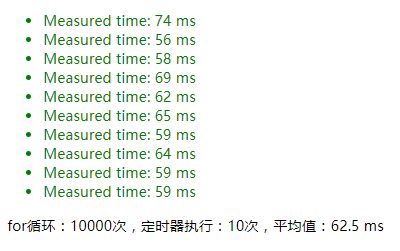2.使用 Object.keys().length 判断一个对象是否为空对象 的结果：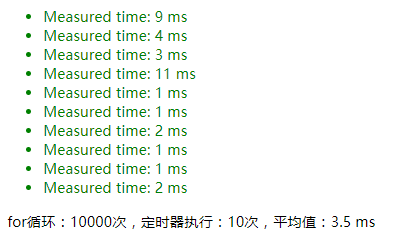maxCount=100000

1.使用 JSON.stringify() 判断一个对象是否为空对象 的结果：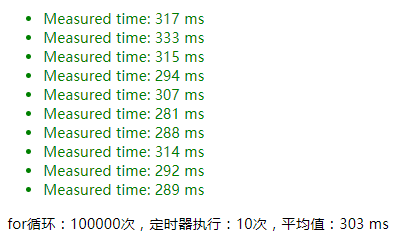2.使用 Object.keys().length 判断一个对象是否为空对象 的结果：